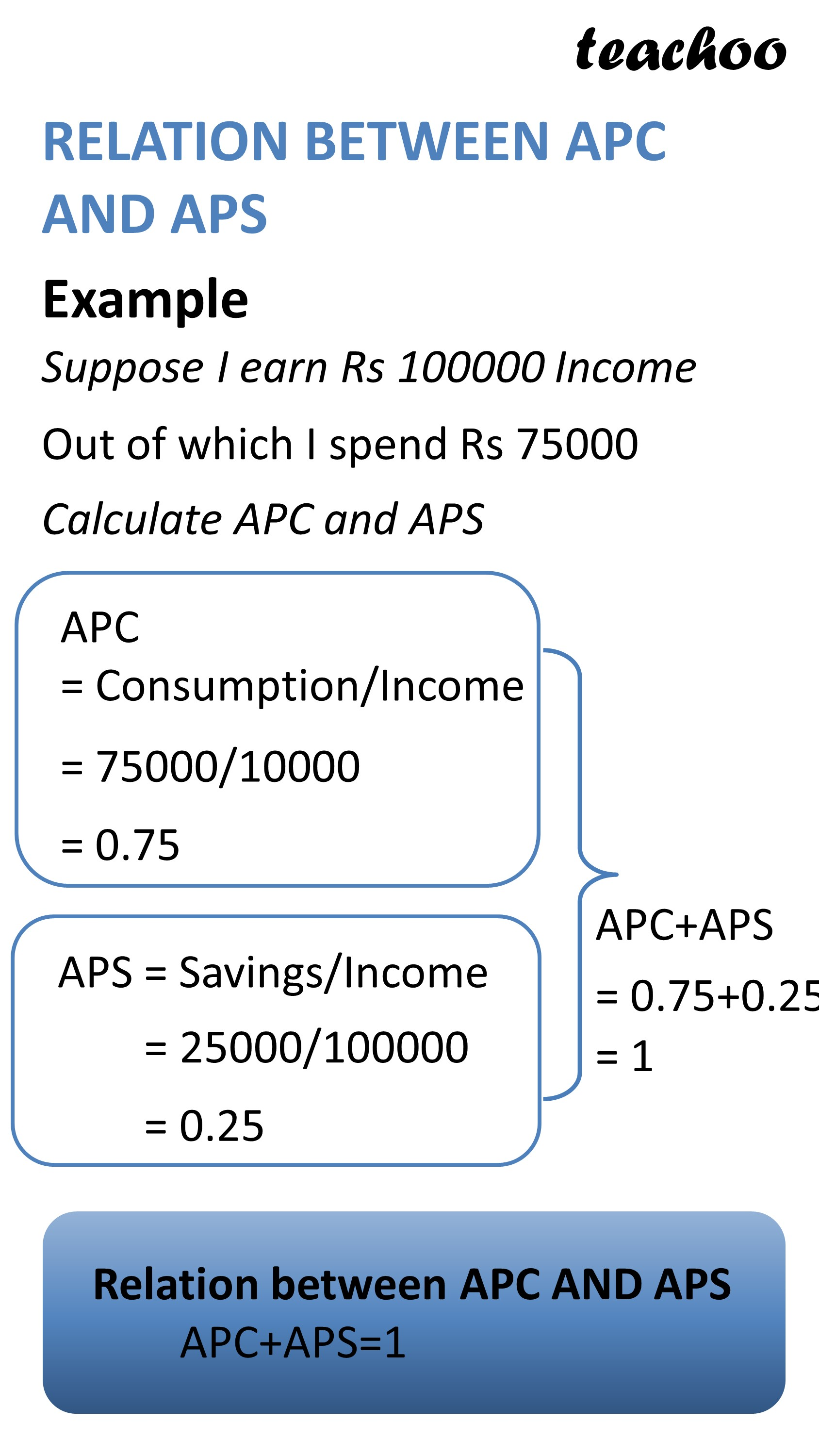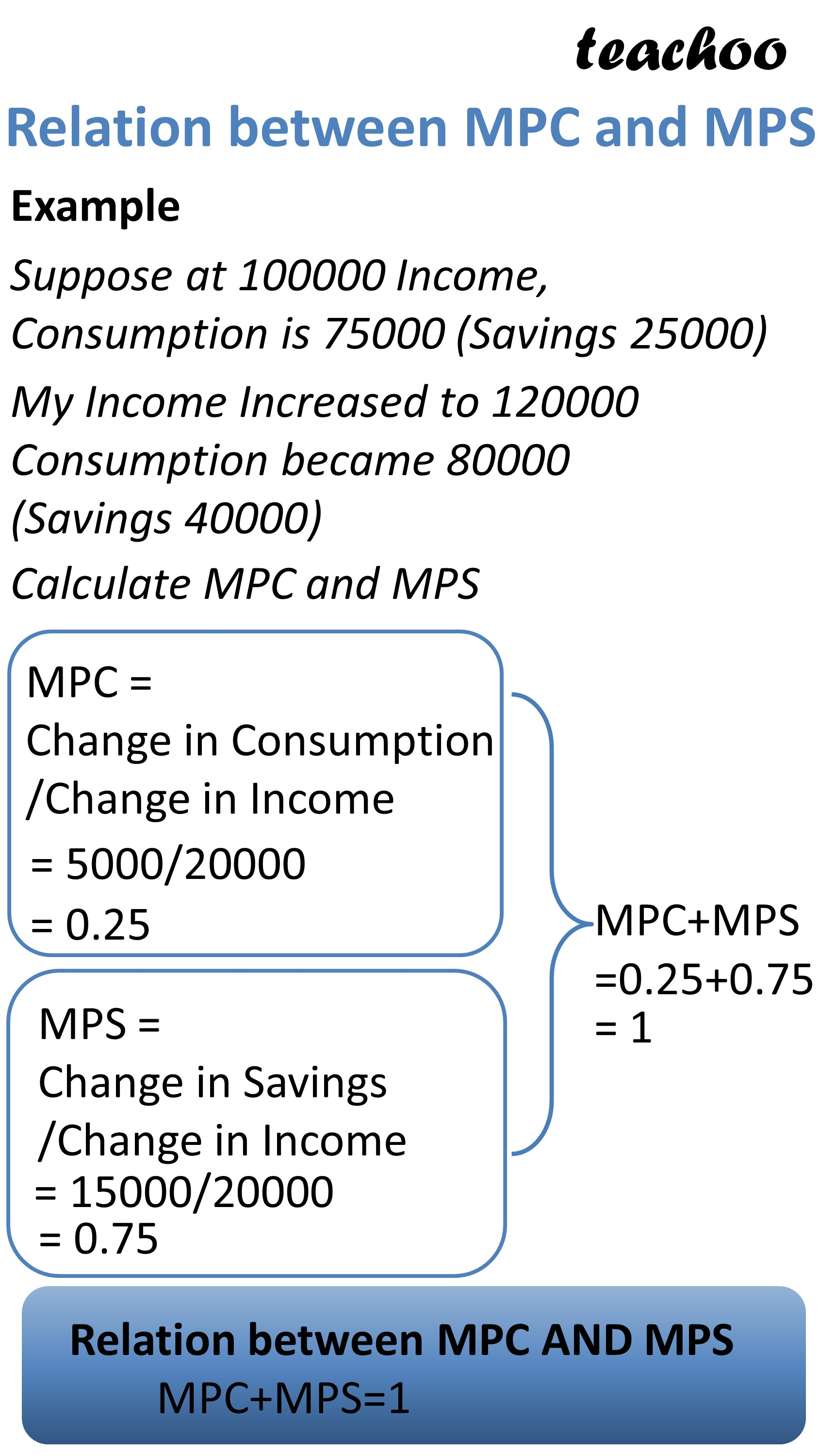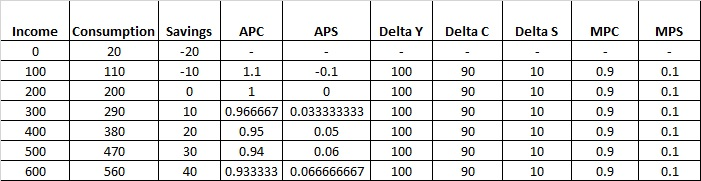Chapter 4 Part 1 - AD,AS and Related Concepts

Economics Class 12
Macroeconomics

APC + APS = 1

MPC + MPS = 1lets understand it with the help of an example

Example 1

Suppose at 100 Income, Consumption is 75

Income increased to 120 and consumption became 90

Calculate Savings, APC, APS, MPC, MPS

Lets first calculate APC and APS

 Income (Y) Consumption Exp Savings APC (C/Y) APS (S/Y) APC + APS 100 75 25 0.75 0.25 1

It can be seen that APC+APS=1

Now, let's calculate MPC and MPS

 Income (Y) Consumption Exp Savings Increase in Income (Delta Y) Increase in Consumption Exp (Delta C) Increase in Savings  (Delta S) MPC (Delta C/Delta Y) MPS (Delta S/Delta Y) MPC + MPS 120 90 30 20 15 5 0.75 0.25 1

It can be seen that MPC+MPS=1

Example 2 Sandeep Garg Page 7.13

Suppose Income and Consumption are as follows

 Income Consumption Savings 0 20 -20 100 110 -10 200 200 0 300 290 10 400 380 20 500 470 30 600 560 40

Calculate APC, APS, MPC, MPSExample 2

If APC of an economy is 0.8, what should be saving at an income level of ₹ 2,000 crores?

APC = 0.8

Income (Y) = 2000

Find Savings?

### NCERT Questions

No questions in this part

#### Question 1

From the following schedule, compute APC, APC MPC and MPS:

 Income 200 250 300 350 400 Saving … 5 15 20 50

#### Question 2

If national income is Rs 50 crore and Saving Rs 5 crore, find out average propensity comsume.

When income rises to Rs 60 crores and saving to Rs 9 crore, what will be the average propensity

to consume and the marginal propensity to save?

Learn in your speed, with individual attention - Teachoo Maths 1-on-1 Class

### Transcript

RELATION BETWEEN APC AND APS Example Suppose I earn Rs 100000 Income Out of which I spend Rs 75000 Calculate APC and APS APC = Consumption/Income = 75000/10000 = 0.75 APS = Savings/Income = 25000/100000 = 0.25 Relation between APC AND APS APC+APS=1 APC+APS = 0.75+0.25 = 1 Reason why APC+APS=1 We know that Income=Consumption + Savings Y=C+S Dividing both sides by Y Y/Y=C/Y+S/Y 1=C/Y+S/Y 1=APC+APS APC+APS=1 Relation between MPC and MPS Example Suppose at 100000 Income, Consumption is 75000 (Savings 25000) My Income Increased to 120000 Consumption became 80000 (Savings 40000) Calculate MPC and MPS MPC = Change in Consumption /Change in Income = 5000/20000 = 0.25 MPS = Change in Savings /Change in Income = 15000/20000 = 0.75 Relation between MPC AND MPS MPC+MPS=1 MPC+MPS =0.25+0.75 = 1 Reason why MPC+MPS=1? We know that Income=Consumption + Savings Change in Income=Change in Consumption + Change in Savings ΔY=ΔC + ΔS Dividing both sides by ΔY ΔY/ΔY=ΔC/ΔY + ΔS/ΔY 1=ΔC/ΔY + ΔS/ΔY 1=MPC+MPS MPC+MPS=1